UNIFORM NETWORKS IN 3-DIMENSIONAL SPACE By: Prof. Michael Burt - Technion, I.I.T-Israel Introduction Abstract and physical 3-D space is not a passive vacuum. It is populated with inter-relating and inter-connected entities, generating assemblages represented as diagrams with a network characteristics and hyperbolic 'force fields', aptly described as sponge surfaces configurations. Diagrams of this kind can represent the structure of almost any plurality that may exist; from a reality of any battle field, cultural economical or political, to transportation and communication systems, to social patterns, cosmological arrays and patterns of perception, knowledge and thought . All networks come in dual pairs. Each network is uniquely determined by, and is a reciprocal of its dual (complementary) companion (Figs.1, A-1). A periodic ordered network is formed by extended repetition of a locally symmetrical association of vertex figures. The resulting configuration of vertices and axes-edges could be described as a polyhedral network, with edges terminated at one or two vertices, each, and vertices joining n-edges together, featuring its bonding valency, so as to conform with the following relation: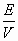=, with E: V&Val.av. standing for the number of Edges, Vertices and average Valency value in a vertex, respectively. In the case of a periodic network, the same applies to its translation unit (T.U.):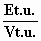=-Every dual pair of networks is associated with a continuous hyperbolical sponge surface which subdivides the space between the two, into two complementary sub-spaces. This trinity of the dual pair and the associated-reciprocal sponge surface is the most conspicuous, all pervading geometric-topological phenomenon of our 3-D space, associated with its order and organization and more than anything else determines the way we perceive and comprehend its structure (Figs. 2, A-2). By defining as 'morphic' those processes which display a movement toward greater 3-dimensional spatial order, symmetry or form (Whyte-1969) and morphology as the logical preoccupation with and manipulation of those processes, than the research into the nature of networks and the associated sponge surfaces may be classified as the essence of morphology. -In some deference from the crystallographic convention, when the network is 3-dimensional, we may call it a space lattice. When it represents a mapping of a 2-d manifold (surface), whether spherical, toroidal or hyperbolical, we may call it a grid. - Conventionally, each space lattice determines a cellular close packing of finite polyhedra (the nature of which may have more than one interpretation). Some periodic space lattices lead to a 'self close-packing', by one type of polyhedral cells. By far, most of the polyhedra associated with close packing are 'saddle polyhedra' with hyperbolical polygonal faces . It transpires that the vertex figure characteristics (geometric-symmetrical and topological) of a given network, tightly correspond to the topological-symmetrical characteristics of the close-pack cells of it's dual. By proxy it may be stated that all constituents of a given 'trinity' (the dual networks pair and the associated sponge surface) act under the same topological – Symmetrical regime. -Connectivity value (C) of the two continuous dual network graphs is one and the same for both, and is the same as genus-(g) value of the associated sponge surface: C=L-N+1=g (with L&N as the number of Line-edges and vertex-Nodes respectively . -Each of the sponge surfaces may be mapped with a grid, representing eventually a sponge polyhedron which conforms with the Euler's theorem and formula, stating that: V-E+F=2(1-g), with g ≥ 2 (when V, E, F&g correspond to the number of Vertices, Edges, Faces and the genus value of the 2d-manifold, respectively). -The total average curvature value ∑av. of a vertex region of a sponge polyhedron may be expressed as : ∑av. = 2[1-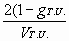], as derived from Descartes' (expanded) theorem, (with VT.U. representing the number of vertices in a translation unit, when the polyhedron is of periodic nature).   Uniform Space Lattices and the associated Periodic Sponge Surfaces and Sponge Polyhedra Any significant venture into the field of periodic sponge surfaces and polyhedra dictates a systematic exploration of the uniform space lattice domain. In stark contrast to the amount of research efforts and energy which was invested over the millennia in the subject of finite (spherical) and toroidal, plane faceted polyhedra, the amount of intellectual attention and exploratory research efforts of the hyperbolical sponge polyhedra domain is incredibly meager. Some explanation may be found in the fact that the overall mathematical-geometrical thinking and imaging was (for almost two millennia) utterly dominated by the Euclidean conception of space which precluded the possibility to discuss Sponge Surfaces and related polyhedra, and also partly because the notion of spatial infinity (and infinite networks and sponge surfaces) was alien to our predecessors, conceptualizing that "everything is finite. Infinite is only the grace of God" (Thomas Mann). And still, it came as a shocking surprise to realize that in spite of the great efforts of the last three centuries or so, in the exploration of the structure of matter and space (crystallography included), no systematic effort was committed to exhaustively explore the network domain in the "abstract realm of the theoretically imaginable". The starting point of this exploration process of uniform networks in 3-D space goes with the preconception (to be tested along the way) that their possible valancy values are in the range of 3÷12 (?!) and therefore it is plausible that the total number of such topologically distinct networks is finite and exhaustive in extent. In this context: Anything less than Val.=3 does not lead to a construction of a space lattice, and – There cannot be anything of a higher valency value than the dodecavalent Octet (close-packing of 3+3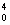) space lattice (?!). Valency appears to be the most conspicuous and domineering characteristic of the 3-dimensional uniform networks, but appropriate attention should be given to few other all-pervading parameters: Spatial density (in terms of a/a3) of the space lattice (the number of edges of the length of, a per cubic volume of a3). As a referential basis we should have in mind that the spatial density of the tetravalent diamond lattice is ~ 1,299a/a3; that of the hexavalent cubic lattice is 3,000 a/a3 and that of the dodecavalent octet lattice is ~ 8,485 a/a3 (By density we refer to the lowest possible value for a distinct topology). It is of great theoretical interest and probably even of practical importance how far down and up can the density values descend or aspire. Network's graph connectivity (C) or the genus (g) of the associated sponge surface values, as featuring in the translation unit of the configuration (CT.U. & g T.U.). When considering the derived sponge surface and the related sponge polyhedra, their overall curvature (expressed in terms of ∑α. In a polyhedral vertex) in a repeating vertex region. The ∑ α is in direct proportion to g T.U. and inversely to VT.U. and is of a great descriptive importance. Although it is still too early to establish all the possible interrelations, it seems that these parameters (Val.; Den.; CT.U. or gT.U. and ∑ α,) are capturing the essence of the related topological-geometrical phenomenon. An assumption is formed that we are dealing with probably not more than few hundreds of uniform space lattices in 3-D space and in view of the valency limiting values and symmetry constraints it seems that an exhaustive systematic search of these configurations is tenable. At the moment of this reporting the task is still far from completion. As a meaningful probe into the realm of the 'theoretically imaginable', it is decided to venture at the bounding periphery of the domain, namely at the trivalent [Figs. 3] and the dodecavalent lattice configurations and possibly at their least and up most density networks [Figs. 4]. Uniform Trivalent Space Lattices A.F. Wells in his monumental work on Structural Inorganic Chemistry (-1962) started that…."The theory of these nets does not appear to be known and in fact no attempt to derive them systematically seems to have been made until comparatively recently (P.100). As a result of these "recent" attempts he lists three, 3-D 3- connected nets... "in which all the smallest circuits are 10-gons"… One of these was announced again by A.F. Wells in 1977 and rediscovered by Toshikazu Sunada (Feb. notices of the American Mathematical society – 2008). [1,6]. Looking into the issue (Jan-July. 2008) it was quite surprising to discover 18 uniform trivalent space lattices, the three a.m. independently rediscovered and included. The attached illustrations Figs.3 show the prime networks (mostly without the duals), the close packing units (mostly saddle polyhedra) and the related sponge surfaces and some associated uniform sponge polyhedra. All spatial densities are bellow 1.o a/a3, with an impressive low density record of 0,254558441 a/a3. with octagonal and 14-gonal circuits. A tabular display (Figs. 5) of the 18 3-valent networks in their order of increasing density, shows that the range of connectivity or genus values (per translation unit) is 3÷25; and the curvature of a vertex region on the resulting sponge surfaces are ∑α=22/3π÷4π.   Uniform Dodecavalent and higher valency Space Lattices or: how far valency and spatial density values can go. Uniform dodecavalent 'octet' based space lattices resulting from close packing of 3+3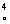(or identical spheres)exist in more than one topological version, but all come to same spatial density of 8,485281374 a/a3. It came as a slight surprise to realize that the infinite sponge polyhedron 3gives rise to a uniform dodecavalent (12-34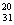) space lattice, the density of which is 10,73918545a/a3 (!) (Figs. 4, H-7). Even the density of the uniform 9-valent (9-314.42.5543) space lattice, representing the 394 infinite sponge polyhedron, is reaching above that of the octet lattice, to 9,72299247a/a3 (!) (Figs. 4, H-1). The quest for higher density networks led to a spatial experiment as follows: Lets perform an edge-length translation of a given uniform n-valent space lattice in an arbitrary direction from position A into position B. The resulting network is a 4-dimensional feature, the 3-dimensional representation of which displays an entangled (interpenetrating) behaviour. The A+B network is (n+1)- valent and a uniform lattice as well. The spatial density of the resulting space lattice will be: Den. (n+1) =(2ET.U.+1), with ET.U. as the number of edges within the translation unit of the lattice. - Two interpenetrating double layer uniform 9-valent and octet based space trusses, when joined by an infinite set of parallel edges, results in a new uniform 11-valent (11-315.41810) space lattice, with a density of 12,70170592a/a3 (Figs. 4, H-9). -Two interpenetrating uniform octet-based dodecavalent space lattices (with edge-length of a) when joined translation wise by an infinite set of parallel edges (of length) a result in a Uniform 13-valent (13-324.41212) space lattice, with a density of 18.384776319a/a3 (!) (Figs. 4, H-10). Few more examples are attached [Figs. 4, H-(1÷10)]. - By connecting two such 13-valent (octet-based) space lattices as previously and repeating the process m times, we will end up with a uniform (n+m)- valent space lattice with a spatial density of : Den. (n+m) = [6x2m +]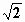a/a3. It could be performed with any other uniform base lattice. In fact m and the spatial density value can reach to infinity (!) and that, at least theoretically, without causing any edge intersection.   Uniform Entangled Space Lattices The uniform interpenetrating 'translation networks' represent a novel feature, unaccounted and unexplored which raises many fundamental theoretical questions. It seems that such networks could not be described as close-packing cellular systems, since in any of these 'translation networks' two independent cellular systems are entangled together. It makes the construction of a conventially defined dual network impossible. It is probable that if the 'translation network' (T.N.), resulting from and edge-length translation of a given uniform n-valent lattice (K) is considered to be a 4-dimensional, no problems arise. The dual of T.N. may be an edge-length translation of the dual of K, and the associated partition surface in 3-d space as its edge-length translation (whatever that means). The difficulty is presented when the translation network and its interpretation is confined to 3-d space. Together, the uniform entangled lattice-T.N and its dual lattice, entangled as well, when superimposed, represent a mess. The subdividing hyperbolical space partition is impossibility, and therefore, represents a mystery. There is no trinity any more. It was found that two uniform trivalent lattices have similar characteristics (Figs. 2,  B-8 &B-9) in the Table B-19, are entangled but of a different genetic order. To all appearances, the number of uniform entangled (n+m) - valent space lattices is reaching to infinity. Uniform Quasi-crystalline space lattices The term Uniform quasi-crystalline space lattice implies a contradiction and in a more precise manner it means – space lattices with locally repeated pentagonal – icosahedral symmetry behavior while globally sustaining one of the crystallographic symmetry groups. The paper present few developed cases, all of which start with an icosahedral base-configuration and by utilizing it's associated tetrahedral, cubic or octahedral symmetries, lead to the construction of uniform space lattices, with a periodic local relation to the icosahedral (finite) symmetry group, while globally behaving in conformity with either the diamond, the 'cubic' or the 'cube-centered' symmetry regime (Figs. 7, K-1;2;3).   In Conclusion The formulation of this paper started while the research of the topic was still incomplete and therefore, its presuppositions were revised and even altered toward the end of its delivery. Networks in the 3-dimensional space in general, and uniform space lattices in particular, are an essential aspect of structural morphology which is mostly concerned with the structure of space and space structures. In particular, any progress in the research of the fields of uniform sponge polyhedra and their genetic sponge surfaces accounting for the greater majority of shapes and forms in nature and in the abstract realm of the theoretically imaginable, strongly depend on the development and generation of uniform space lattices. In conclusion, the networks in 3-D space represent two basically different phenotypes: Networks which could be characterized as consisting of a trinity of spatially associated features of a dual pair of space lattices and the reciprocal hyperbolic surface partition, subdividing the space between the two, The entangled networks, in themselves representing few classes, the nature of their duals and the associated partition surfaces are still to be explored. Concerning the 'Trinity Networks' (1), on the basis of their symmetry constraints in 3-d space and the resulting valency range in a vertex (Val.=3÷12), a conclusion was drawn that their domain of all 'theoretically imaginable' uniform space lattices is limited in scope to the extent that enables their exhaustive systematic identification and enumeration. So far nearly 180 such uniform space lattices were identified with their density ranging from 0,2545 a/a3 (for the trivalent) to 10,7392 a/a3 (for the dodecavalent) (Figs.2, Figs.3, Figs.4, Figs.5, Figs.6). The Uniform Entangled Space Lattices, first encountered as uniform trivalent lattices (density of 0,4375 a/a3), were mostly generated as 'translation networks', by moving a uniform n-valent lattice by and edge-length distance, to result in a uniform entangled (n+1)-valency lattice. While the lower valency and density boundaries are quite definite and tangible the upper limits can reach to infinity. Within the specific valency category, no particular binding relations are discernible to exist between the density; the CT.U. (or g T.U. ) values and not between g T.U.; ∑α or Val. Values of related sponge polyhedra, although on the macro-scale of the lattice valency evolution it is possible to state that most of the parameters mentioned above (density included), do increase with the rising lattice valency values. References: A.F.Wells-(1962), 'Structural Inorganic Chemistry' Oxford Univ. Press, G.B A.F.Wells-(1977), 'Three Dimensional Nets and Polyhedra' John Wiley and sons Inc. A. Judge – (2008), 'Polyhedral Empowerment of Networks Through Symmetry: Psycho: Social Implications' J.Kappraff – (1991), 'Connections, The Geometric, Bridge Between Art And Science' McGraw Hill, Inc. Whyte, Wilson & Wilson – (1969), 'Hierarchical Structures' American Elsevier Publishing Company, Inc. Scientific American (March – 2008) Crystal Math. – page 19. M.Burt – (1966), 'Spatial Arrangement And Polyhedra With Curved Surfaces And Their Architectural Application'. Technion Publication, Israel Wachman, Burt, Kleinman – (1974, 2005), 'Infinite Polyhedra' Technion Publication, Israel. M.Burt – (1996), 'The periodic Table Of The Polyhedral Universe'. Technion Publication, Israel M.Burt – (2007), 'Periodic Sponge Surfaces And Uniform Sponge Polyhedra In Nature And In The Realm Of The Theoretically Imaginable' VISMATH – http://www.mi.sanu.ac.yu/vismath